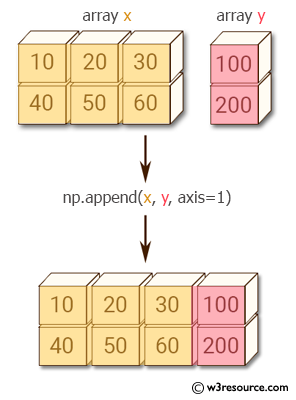﻿ NumPy: How to add an extra column to a NumPy array - w3resource# NumPy: How to add an extra column to a NumPy array

## NumPy: Array Object Exercise-86 with Solution

Write a NumPy program to add an extra column to a NumPy array.

Pictorial Presentation:Sample Solution:-

Python Code:

``````import numpy as np
x = np.array([[10,20,30], [40,50,60]])
y = np.array([, ])
print(np.append(x, y, axis=1))
```
```

Sample Output:

```[[ 10  20  30 100]
[ 40  50  60 200]]
```

Python Code Editor:

Have another way to solve this solution? Contribute your code (and comments) through Disqus.

What is the difficulty level of this exercise?

Test your Python skills with w3resource's quiz

﻿

## Python: Tips of the Day

Set comprehension:

```>>> m = {x ** 2 for x in range(5)}
>>> m
{0, 1, 4, 9, 16}
```